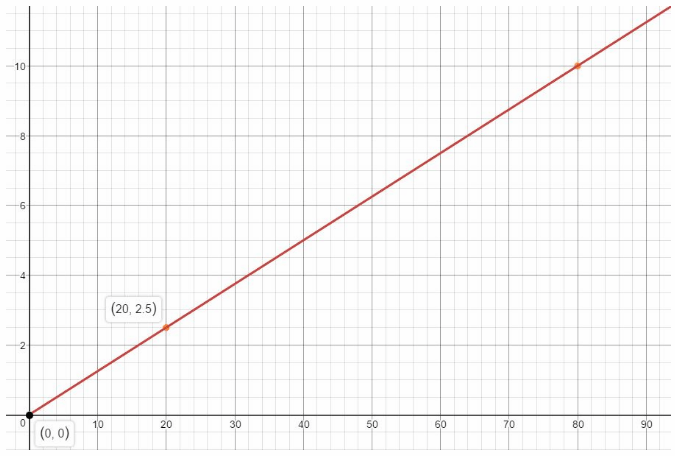# Texas Go Math Grade 6 Lesson 8.3 Answer Key Solving Problems with Proportions

Refer to our Texas Go Math Grade 6 Answer Key Pdf to score good marks in the exams. Test yourself by practicing the problems from Texas Go Math Grade 6 Lesson 8.3 Answer Key Solving Problems with Proportions.

## Texas Go Math Grade 6 Lesson 8.3 Answer Key Solving Problems with Proportions

Question 1.
The PTA is ordering pizza for their next meeting. They plan to order 2 cheese pizzas for every 3 pepperoni pizzas they order. How many cheese pizzas will they order if they order 15 pepperoni pizzas?
$$\frac{\text { cheese }}{\text { pepperoni }}=\frac{2}{3}=\frac{x}{15}$$
15 is a common denominator:
$$\frac{2}{3} \cdot \frac{5}{5}=\frac{x}{15}$$
$$\frac{10}{15}=\frac{x}{15}$$
⇒ x = 10
10 cheese pizzas.

Question 2.
Ms. Reynold’s sprinkler system has 9 stations that water all the parts of her front and back lawn. Each station runs for an equal amount of time. If it takes 48 minutes for the first 4 stations to water, how long does it take to water all parts of her lawn? _______________
It takes 48 minutes for the first l stations to water. This implies that each station takes $$\frac{48}{4}$$ = 12 minutes. Therefore the total of 9 stations will require a total time of 9 × 12 = 108 minutes.

9 stations will require 108 minutes.

Question 3.
The distance between Sandville and Lewiston is shown on the map. What is the actual distance between the towns? ___________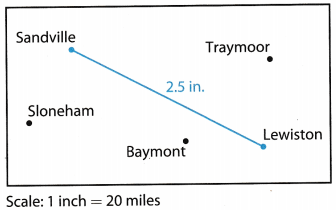Write a proportion.
$$\frac{20 \text { miles }}{1 \text { inch }}=\frac{? \text { miles }}{2.5 \text { inches }}$$ write the scale as a unit rate
Write an equivalent rate to find the missing number
$$\frac{20 \text { miles } \times 2.5}{1 \text { inch } \times 2.5}=\frac{50 \text { miles }}{2.5 \text { inches }}$$
So, the missing number is 50.
The actual distance between the two towns is 50 miles
Final Solution = 50

Find the unknown value in each proportion.

Question 1.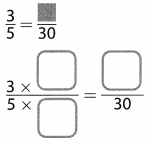Write a proportion
$$\frac{3}{5}=\frac{x}{30}$$
Use common denominators to write equivalent ratio&
$$\frac{3 \times 6}{5 \times 6}=\frac{x}{30}$$
30 is a common denominator

$$\frac{18}{30}=\frac{x}{30}$$
Equivalent ratios with the same denominators have the same numerators
x = 18
The unknown value is 18
Final solution = 18

Question 2.Write a proportion.
$$\frac{4}{10}=\frac{x}{5}$$
Use common denominators to write equivalent ratios.
$$\frac{4 \div 2}{10 \div 2}=\frac{x}{5}$$ 10 is a common denominator

$$\frac{2}{5}=\frac{x}{5}$$
Equivalent ratios with the same denominators have the same numerators
x = 2
The unknown value is 2
Final solution = 2

Solve using equivalent ratios.

Question 3.
Leila and Jo are two of the partners in a business. Leila makes $3 in profits for every$4 that Jo makes. If Jo makes \$60 profit on the first item they sell, how much profit does Leila make?
Write a proportion.
$$\frac{\text { Leila’s profit }}{\text { Jo’s profit }}=\frac{3}{4}=\frac{?}{60}=\frac{\text { Leila’s profit }}{\text { Jo’s profit }}$$
Use common denominators to write equivalent ratios.
$$\frac{3 \times 15}{4 \times 15}=\frac{?}{60}$$ 60 is a common denominator

$$\frac{45}{60}=\frac{?}{60}$$
Equivalent ratios with the same denominators have the same numerators

? = 45
If Jo makes 60 dollars profit Leila makes 45 dollars profit
Final solution = 45

Question 4.
Hendrick wants to enlarge a photo that is 4 inches wide and 6 inches tall. The enlarged photo keeps the same ratio. How tall is the enlarged photo if it is 12 inches wide?
Write a proportion.
$$\frac{6}{4}=\frac{x}{12}$$
Use common denominators to write equivalent ratios.
$$\frac{6 \times 3}{4 \times 3}=\frac{x}{12}$$ 12 is a common denominator

$$\frac{18}{12}=\frac{x}{12}$$
Equivalent ratios with the same denominators have the same numerators

x = 18
The unknown value is 18
The enlarged photo is 18 inches tall
Final Solution = 18

Solve using unit rates.

Question 5.
A person on a moving sidewalk travels 21 feet in 7 seconds. The moving sidewalk has a length of 180 feet. How long will it take to move from one end to the other?
Evaluate the unit rate of the distance traveled. Here it is $$\frac{21}{7}$$ = 3 feet per second. This implies that the time required to cover the moving walkway 180 feet long is $$\frac{180}{3}$$ = 60 seconds.

It takes 60 seconds to move from one end of the sidewalk to the other.

Question 6.
In a repeating musical pattern, there are 56 beats in 7 measures. How many measures are there after 104 beats?
To find the unit rate, divide the numerator and denominator by 7: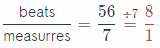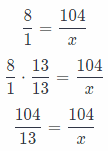x = 13
There are 13 measures.

Question 7.
Contestants in a dance-a-thon rest for the same amount of time every hour. A couple rests for 25 minutes in 5 hours. How long did they rest in 8 hours?
To find the unit rate, divide the numerator and denominator by 5:⇒ x = 40
They rested for 40 minutes.

Question 8.
Francis gets 6 paychecks in 12 weeks. How many paychecks does she get in 52 weeks?
To find the unit rate, divide the numerator and denominator by 12: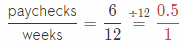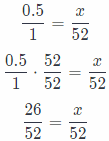⇒ x = 26

Question 9.
What is the actual distance between Gendet and Montrose? ________________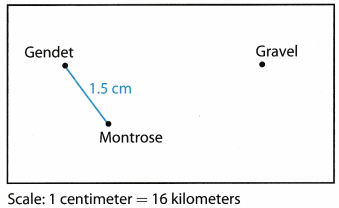Write a proportion.
$$\frac{16 \mathrm{~km}}{1 \mathrm{~cm}}=\frac{? \mathrm{~km}}{1.5 \mathrm{~cm}}$$ Write the scale as a unit rate

Write an equivalent rate to find the missing number
$$\frac{16 \mathrm{~km} \times 1.5}{1 \mathrm{~cm} \times 1.5}=\frac{24 \mathrm{~km}}{1.5 \mathrm{~cm}}$$
So, the missing number is 24

The actual distance between the two towns is 24 kilometers
Final solution = 24

Essential Question Check-In

Question 10.
How do you solve problems with proportions?
Problems with proportions are converted to an equation using the given situation and then basic laws of algebra are used to evaluate the unknown quantity ¡n the given proportion.

Question 11.
On an airplane, there are two seats on the left side in each row and three seats on the right side. There are 90 seats on the right side of the plane.
a. How many seats are on the left side of the plane? _______________
There are 3 seats on the right side and there are 90 seats on the right side of the plane This implies that there are $$\frac{90}{3}$$ = 30 rows in the plane. There are 2 seats on the left side plane, so in total there are 30 × 2 = 60 seats on the left hand side of the plane.

b. How many seats are there altogether? _______________
There are a total of 90 + 60 = 150 seats on the plane.

Question 12.
The scale of the map is missing. The actual distance from Liberty to West Quail is 72 miles, and it is 6 inches on the map.a. What is the scale of the map?
The actual distance from liberty to West Quail is 72 miles, and it ¡s 6 inches on the map. This means that each represents an actual distance of $$\frac{72}{6}$$ = 12 miles, so the scale is 1 inch = 12 miles.

b. Foston is directly between Liberty and West Quail and is 4 inches from Liberty on the map. How far is Foston from West Quail? Explain.
Foston s between Liberty and West Quail and is 4 inches from Liberty on the map This impLies that Foston is 6 – 4 = 2 inches away from West Quall on the map. Therefore the actual distance between Foston and West Quall is 2 × 12 = 24 miles.

Question 13.
Wendell is making punch for a party. The recipe he is using says to mix 4 cups pineapple juice, 8 cups orange juice, and 12 cups lemon-lime soda in order to make 18 servings of punch.

a. How many cups of punch does the recipe make? _______________
It makes: 4 pineapple – 8 orange + 12 Lemon-Lime = 24 cups

b. If Wendell makes 108 cups of punch, how many cups of each ingredient will he use?
__________ cups pineapple juice
__________ cups orange juice
__________ cups lemon-lime soda
To find the unit rate, divide the numerator and denominator by 6:108 is a common numerator:
$$\frac{4}{1} \cdot \frac{27}{27}=\frac{108}{x}$$
$$\frac{108}{27}=\frac{108}{x}$$
⇒ x = 27
27 cups pineapple juice

To find the unit rate, divide the numerator and denominator by 8:108 is a common numerator:
$$\frac{3}{1} \cdot \frac{36}{36}=\frac{108}{x}$$
$$\frac{108}{36}=\frac{108}{x}$$
⇒ x = 36
36 cups orange juice

To find the unit rate, divide the numerator and denominator by 12: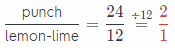108 is a common numerator:
$$\frac{2}{1} \cdot \frac{54}{54}=\frac{108}{x}$$
$$\frac{108}{54}=\frac{108}{x}$$
⇒ x = 54
54 cups lemon-lime juice

c. How many servings can be made from 108 cups of punch? __________
a. 24 cups

b. 27 cups pineapple juice
36 cups orange juice
54 cups Lemon-Lime juice

Question 14.
Carlos and Krystal are taking a road trip from Greenville to North Valley. Each has their own map, and the scales on their maps are different.

a. On Carlos’s map, Greenville and North Valley are 4.5 inches apart. The scale on his map is 1 inch = 20 mîles. How far is Greenville from North Valley?
The scale on is 1 inch = 20 miles, so a scale distance of 4.5 inches imply an actual distance of 4.5 × 20 = 90 miles. Therefore, it can be said that Greenville and North Valley are 90 miles apart.

b. The scale on Krystal’s map is 1 inch = 18 miles. How far apart are Greenville and North Valley on Krystal’s map?
The scale on is 1 inch = 18 miles, so a total distance of 90 miles imply an scale distance of $$\frac{90}{18}$$ = 5 inches on the map Therefore, it can be said that Greenville and North Valley are 5 inches apart on Krystal’s map

Question 15.
Multistep A machine can produce 27 inches of ribbon every 3 minutes. How many feet of ribbon can the machine make in one hour? Explain.
A machine can produce 27 inches of ribbon every 3 minutes. This implies that the rate of ribbon production is $$\frac{27}{3}$$ = 9 inches per minute. Therefore, in 1 hour which consists of 60 minutes, 60 × 9 = 540 inches of ribbon will be made.

540 inches of ribbon will be made in 1 hour.

Marta, Loribeth, and Ira all have bicycles. The table shows the number of miles of each rider’s last bike ride, as well as the time it took each rider to complete the ride.Question 16.
What is Marta’s unit rate, in minutes per mile? __________
Martas unit rate, in minutes per mile is equal to $$\frac{80}{8}$$ = 10.

Martas unit rate is equal to 10 minutes per mile

Question 17.
Whose speed was the fastest on their last bike ride? ______________
Evaluate the speed of each rider by dividing the distance traveled by the time taken. Therefore, Marta’s speed is equal to $$\frac{8}{80}$$ = 0.1 miles per minute. Loribeth’s speed is equal to $$\frac{6}{42}$$ = 0.143 miles per minute and Ira’s speed is equal to $$\frac{15}{75}$$ = 0.2 miles per minute. Since 0.2 > 0.143 > 0.1, Ira’s speed was the fastest

Question 18.
If all three riders travel for 3.5 hours at the same speed as their last ride, how many total miles will all 3 riders have traveled? Explain.
Evaluate the speed of each rider by dividing the distance traveled by the time taken. Therefore, Marta’s speed is equal to $$\frac{8}{80}$$ = 0.1 miles per minute. Loribeth’s speed is equal to $$\frac{6}{42}$$ = 0.143 miles per minute and Ira’s speed is equal to $$\frac{15}{75}$$ = 0.2 miles per minute. Since 0.2 > 0.143 > 0.1, Ira’s speed was the fastest.

3.5 hours is equal to 3.5 × 60 = 210 minutes. Therefore in 210 minutes, Marta would have covered a distance of 0.1 × 210 = 21 miLes, Loribeth would have covered a distance of 0.143 × 210 = 30 miles and Ira would have covered a distance of 0.2 × 210 = 42 miles. Altogether they would have covered a distance of 21 + 30 + 42 = 93 miles.

Altogether they would have covered a distance of 93 miles in 35 hours.

Question 19.
Critique Reasoning Jason watched a caterpillar move 10 feet in 2 minutes. Jason says that the caterpillar’s unit rate is 0.2 feet per minute. Is Jason correct? Explain.
Jason watched a caterpillar move 10 feet in 2 minutes, so its unit rate is $$\frac{10}{2}$$ = 5 feet per minute and not 0.2 feet per minute.

H.O.T. Focus On Higher Order Thinking

Question 20.
Analyze Relationships If the number in the numerator of a unit rate is 1, what does this indicate about the equivalent unit rates? Give an example.
The word unit rate implies that the denominator is equal to 1. If the numerator is also equal to 1, then this implies that the 2 quantities are equal and so their equivalent rates will always be equal to 1 An example of this is a speed of a bicycle rider who covers a distance of 5 meters in 5 seconds, so his unit rate or speed will be = 1 meter per second.

Question 21.
Multiple Representations A boat travels at a constant speed. After 20 minutes, the boat has traveled 2.5 miles. The boat travels a total of 10 miles to a bridge.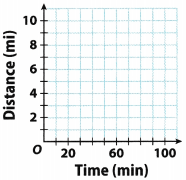a. Graph the relationship between the distance the boat travels and the time it takes.